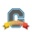## How will I use a formula question with exponents?Community Explorer

Hello everyone.  I was trying to use formula question for my quiz that includes this formula.  a2 + b2 = c2.

My challenge is that I cannot encode the formula inside the Formula Box under Formula Definition.  How will I do it?

Thank you.

1 SolutionCommunity Coach

Hi there,  @glenda_obre ...

I'm not sure if this is a full answer for you, but for your formula box, could you try something like: (a^2)+(b^2) ?